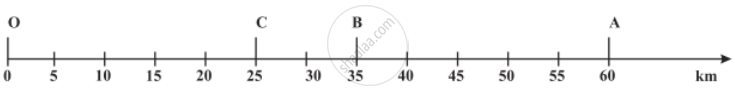# Motion Along a Straight Line

• One dimensional motion
• Representation of one dimension notion

## Notes

Motion Along a Straight Line:

When an object moves along a straight line, the motion of the object is called rectilinear motion. For example; motion of a car on highway.

Let A, B and C represent the position of the object at different instants. At first, the object moves through C and B and reaches A. Then it moves back along the same path and reaches C through B.The total path length covered by the object is OA + AC, that is 60 km + 35 km = 95 km. This is the distance covered by the object.

Distance is not dependent upon the direction of the motion.

For motion of the object from O to A, the distance covered is 60 km and the magnitude of displacement is also 60 km. During its motion from O to A and back to B, the distance covered = 60 km + 25 km = 85 km

while the magnitude of displacement = 35 km. Thus, the magnitude of displacement (35 km) is not equal to the path length (85 km). Further, we will notice that the magnitude of the displacement for a course of motion may be zero but the corresponding distance covered is not zero. If we consider the object to travel back to O, the final position concides with the initial position, and therefore, the displacement is zero. However, the distance covered in this journey is OA + AO = 60 km + 60 km = 120 km.

Thus, two different physical quantities — the distance and the displacement, are used to describe the overall motion of an object and to locate its final position with reference to its initial position at a given time.

If you would like to contribute notes or other learning material, please submit them using the button below.